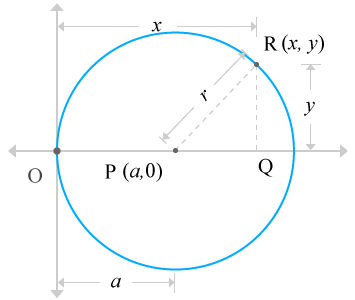# Equation of the Circle when circle passes through the origin and centre of the circle lies on x-axis

Expressing a circle in a mathematical form expression when the circle passes through the origin of Cartesian coordinate system and centre of the circle lies on x-axis is defined Equation of the Circle when circle passes through the origin and centre of the circle lies on x-axis.

Geometrically, a circle can be passed through the origin of the Cartesian coordinate geometric system and also the centre of the same circle may also lie on the horizontal x-axis. It can be expressed in a mathematical equation form in mathematics by understanding the relation of circle with Cartesian coordinate system and it understands us how exactly a circle is, in Cartesian coordinate system.

## Derivation

Consider a circle, whose radius is assumed as r units. Also assumed, the centre of the circle lies on the x-axis and the circle passes through the origin of the Cartesian coordinate system.Assume, the centre of the circle is denoted by a letter P and the point P is located at a units distance from the origin in horizontal x-axis direction but the point is lied on the x-axis. So, the vertical distance of this point P is zero units. Therefore, the coordinates of the point P is P (a, 0).

Consider a point on the circle, called point R which is located at R (x, y). Assume, it is a variable point, which represents each point on the circle.

Draw a line towards horizontal axis from the point R perpendicularly and draw a line from point P on the horizontal axis until both lines get intersected at a point, called Q. This procedure constructed a right angled triangle, known ΔRPQ.

The triangle is a right angled triangle. So, applying Pythagorean Theorem to triangle ΔRPQ gives us relation between three sides of the triangle.

PR2 = PQ2 + RQ2

The values of the each side of the right angled triangle can be evaluated from the right angled triangle ΔRPQ.

• Length of the Opposite side is RQ = y
• Length of the Adjacent side is PQ = OQ  –  OP = x  –  a
• Length of the Hypotenuse is PR = r

Substitute these three values in the mathematical relation, developed from the Pythagorean Theorem.

r2 = (x  –  a)2 + y2

⇒ (x  –  a)2 + y2 = r2

The point P is located at a units distance from the origin on the x-axis. So, the length of the OP is equal to a units; means OP = a. Actually, the point P is centre of the circle and the circle passes through the origin. So, the distance from origin O to point P is known radius of the circle. It means OP = r. Therefore, OP = r = a. As per this rule, the algebraic expression can be written as follows.

(x  –  a)2 + y2 = a2

⇒ x2 + a2  –  2ax + y2 = a2

⇒ x2  –  2ax + y2 = 0

It’s an algebraic equation, which represents a circle when the circle is passing through the origin and also its centre lies on the x-axis.

A best free mathematics education website that helps students, teachers and researchers.

###### Maths Topics

Learn each topic of the mathematics easily with understandable proofs and visual animation graphics.

###### Maths Problems

A math help place with list of solved problems with answers and worksheets on every concept for your practice.

Learn solutions

###### Subscribe us

You can get the latest updates from us by following to our official page of Math Doubts in one of your favourite social media sites.